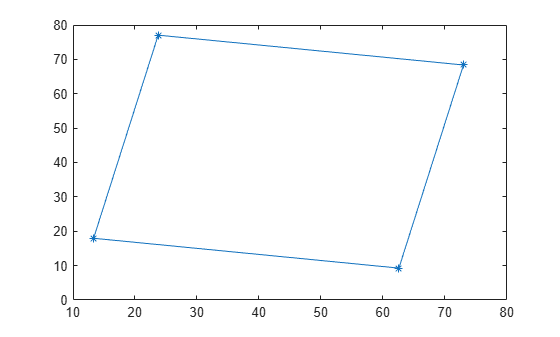# bbox2points

Convert rectangle to corner points list

## Syntax

``points = bbox2points(rectangle)``

## Description

example

````points = bbox2points(rectangle)` converts the input rectangle, specified as [x y width height] into a list of four [x y] corner points. The `rectangle` input must be either a single bounding box or a set of bounding boxes.```

## Examples

collapse all

Define a bounding box.

`bbox = [10,20,50,60];`

Convert the bounding box to a list of four points.

`points = bbox2points(bbox);`

Define a rotation transformation.

```theta = 10; tform = affine2d([cosd(theta) -sind(theta) 0; sind(theta) cosd(theta) 0; 0 0 1]);```

Apply the rotation.

`points2 = transformPointsForward(tform,points);`

Close the polygon for display.

`points2(end+1,:) = points2(1,:);`

Plot the rotated box.

`plot(points2(:,1),points2(:,2), '*-');`## Input Arguments

collapse all

Bounding box, specified as a 4-element vector, [x y width height], or a set of bounding boxes, specified as an M-by-4 matrix.

Data Types: `single` | `double` | `int16` | `int32` | `uint16` | `uint32`

## Output Arguments

collapse all

List of rectangle corners, returned as a 4-by-2 matrix of [x,y] coordinates, or a 4-by-2-by-M array of [x,y] coordinates. The output points for the rectangle are listed counterclockwise starting from the upper-left corner.

• For a single input bounding box, the function returns the 4-by-2 matrix.

• For multiple input bounding boxes, the function returns the 4-by-2-M array for M bounding boxes.

Data Types: `single` | `double` | `int16` | `int32` | `uint16` | `uint32`